Would you like to see this set of cheatsheets in your native language? You can help us translating them on GitHub!
Teaching

### CS 229 ― Machine Learning Star

My twin brother Afshine and I created this set of illustrated Machine Learning cheatsheets covering the content of the CS 229 class, which I TA-ed in Fall 2018 at Stanford. They can (hopefully!) be useful to all future students of this course as well as to anyone else interested in Machine Learning.

#### Cheatsheet

Supervised Learning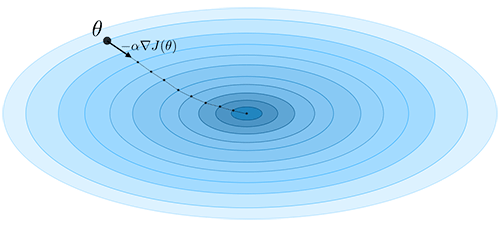• • Loss function, gradient descent, likelihood
• • Linear models, Support Vector Machines, generative learning
• • Tree and ensemble methods, k-NN, learning theory
Unsupervised Learning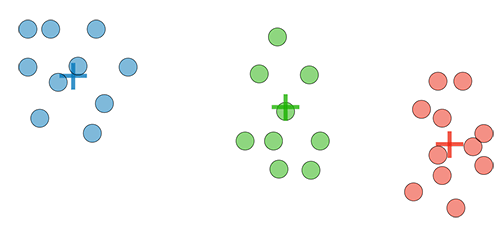• • Expectation-Maximization, k-means, hierarchical clustering
• • Clustering assessment metrics
• • Principal component analysis, independent component analysis
Deep Learning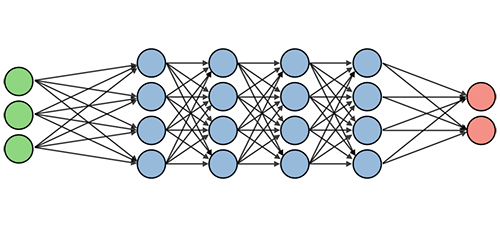• • Architecture, activation function, backpropagation, dropout
• • Convolutional layer, batch normalization, types of gates
• • Markov decision processes, Bellman equation, Q-learning
Tips and tricks• • Confusion matrix, accuracy, precision, recall, F1 score, ROC
• • R squared, Mallow's CP, AIC, BIC
• • Cross-validation, regularization, bias/variance tradeoff, error analysis

#### Refresher

Probabilities and Statistics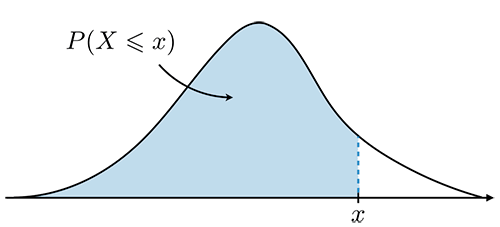• • Axioms of probability, permutation, Bayes' rule, independence, conditional probability
• • Random variable, expectation, variance
• • Central Limit Theorem, parameter estimation
Linear Algebra and Calculus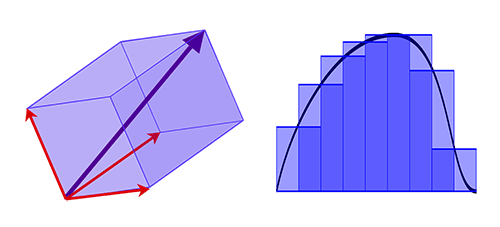• • Main matrices, multiplication, transpose, inverse, trace, determinant
• • Eigenvalue, eigenvector, norm, gradient, Hessian
• • Positive semi-definite matrix, spectral theorem, singular-value decomposition